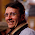## Sunday, February 8, 2009

### Maximum sub-array sum

Given an array of integers, find the sub-array of contiguous elements with maximal sum. In case of ties select the shortest sub-array.

1.I like this problem. The naive solution is exponential. The less naive solution is quadratic, but there is a smart linear solution.

2.About the linear solution. Consider the sub-array with maximum sum [i... j]. then

1. Any sub-array [i... k] with i <= k < j must be positive, otherwise the subarray [k+1 ... j] would have a sum larger than the one of the sub-array [i...j] and this is a contradiction.

2. There exist an l : 1 <= l < i such that the sub-array [l ... i-1] has negative sum, otherwise the sub-array [l ... j] would have a sum larger than the one [i ... j] and this is a contradiction.

As a consequence during a linear scan when the num is negative or zero, we can safely start a new segment

3.@codingplayground

I disagree with your point 1. Consider the following sequence:
1,-15,10,5,5
you can see that the sub-array [k+1...j] = 15 is larger than the sub-array [i..j] = 6

4.[i...j]=6 is not maximum